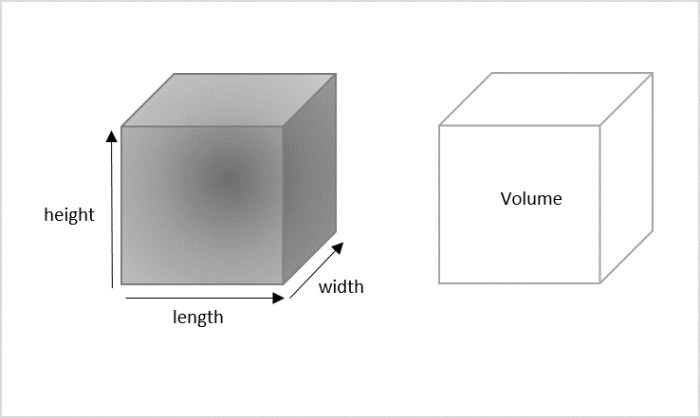# Python Program to calculate the volume of Cube

A cube is a three-dimensional solid figure with six faces, twelve edges and eight vertices. This geometrical figure has equal sized edges, hence making all its dimensions − length, width and height − equal.

The idea of calculating the volume of a cube can be understood in a simple way. Consider a real-time situation where a person a moving houses. Suppose they use a hollow cube shaped cardboard box to place their things in it, the amount of space present to fill it up is defined as the volume.The mathematical formula to calculate the volume of a cube with a side length ‘a’ is as follows −

(a)*(a)*(a)


## Input Output Scenarios

The python program to calculate the volume of the cube will provide the following input-output scenarios −

Assume the length of the cube edge is positive, the output is obtained as −

Input: 6 // 6 is the edge length
Result: Volume of the cube = 216


Assume the length of the cube edge is negative, the output is obtained as −

Input: -6 // -6 is the edge length
Result: Not a valid length


## Using Mathematical Formulae

As seen above, the formula to calculate the volume of a cube is relatively simple. Therefore, we write a python program to display the volume by taking the length of an edge in a cube as an input.

### Example

In the python code below, we calculate the cube volume using its mathematical formula and the length of the cube is taken as the input.

#length of the cube edge
cube_edge = 5

#calculating the volume
cube_volume = (cube_edge)*(cube_edge)*(cube_edge)

#displaying the volume
print("Volume of the cube: ", str(cube_volume))


### Output

The volume of a cube is displayed as the output for the python code above. −

Volume of the cube: 125


## Function to calculate Volume

Another way to calculate volume of a cube in python is using functions. Python has various built-in functions but also has a provision to declare user−defined functions. We use def keyword to declare functions and can pass as many arguments as we want. Mentioning return types is not necessary while declaring python functions.

The syntax to declare a function is −

def function_name(arguments separated using commas)


### Example

In the following python program, the

#function to calculate volume of a cube
def volume(cube_edge):

#calculating the volume
cube_volume = (cube_edge)*(cube_edge)*(cube_edge)

#displaying the volume
print("Volume of the cube: ", str(cube_volume))

#calling the volume function
volume(5) #length of an edge = 5


### Output

On executing the python code above, the output is displayed as −

Volume of the cube: 125